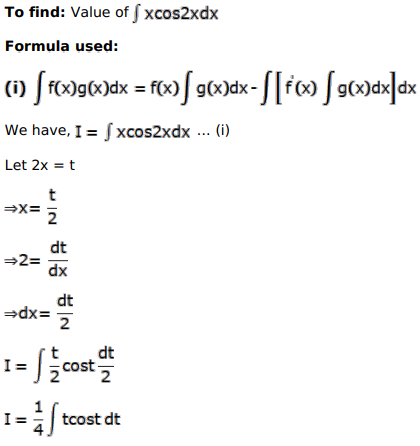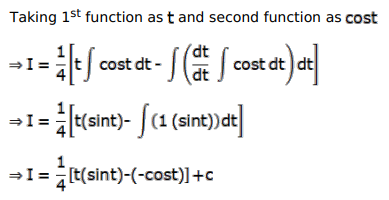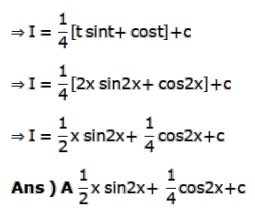# Mark against the correct answer in each of the following:

Question:

Mark $(\sqrt{)}$ against the correct answer in each of the following:

$\int x \cos 2 x d x=?$

A. $\frac{1}{2} x \sin 2 x+\frac{1}{4} \cos 2 x+C$

B. $\frac{1}{2} x \sin 2 x-\frac{1}{4} \cos 2 x+C$

C. $2 x \sin 2 x+4 \cos 2 x+C$

D. none of these

Solution: